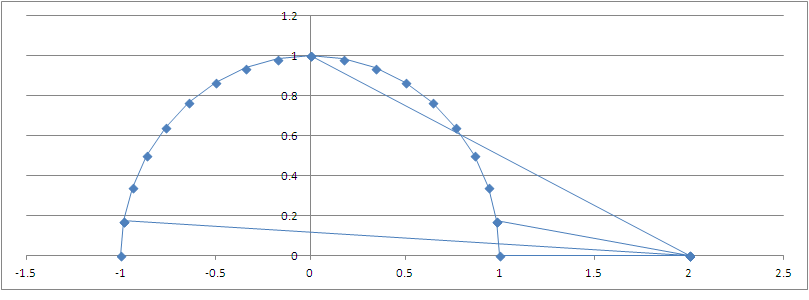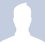# $F$ = $\frac{GMm}{d^2}$Parametric form for $x^2 + y^2 = 1^2$ is $(\cos \theta, \sin \theta).$

$\tan \phi$ = $\displaystyle \frac{\sin \theta}{2 - \cos \theta}$ {$\phi$ is an angle at (2, 0)}

$\implies \cos \phi$ = $\displaystyle \frac{2 - \cos \theta}{\sqrt{5 - 4 \cos \theta}}$

D = $\sqrt{(\cos \theta - 2)^2 + (\sin \theta - 0)^2}$ = $\sqrt{5 - 4 \cos \theta}$

Therefore, D $\cos \phi$ = d = 2 - $\cos \theta$

$\overline{d} = \displaystyle \frac{1}{\pi} \int_0^{\pi} (2 - \cos \theta) d \theta$ = $\displaystyle \frac{1}{\pi} [2 \theta - \sin \theta]_0^{\pi} = 2$

There is nothing wrong with center of gravity to have an average distance of 2 from (0, 0) to (2, 0).

$F$ = $G m$ $\displaystyle \frac{1}{2 \pi}$ $\displaystyle \int_{-\pi}^{\pi} \frac{d M}{d \theta} \frac{d \theta}{d^2}$

$\implies F = G m \frac{d M}{d \theta} \frac{1}{2 \pi}$ $\displaystyle \int_{-\pi}^{\pi} \frac{d \theta}{d^2}$ = $G m$ $\frac{d M}{d \theta} \frac{1}{\pi}$ $\displaystyle \int_{0}^{\pi} \frac{d \theta}{(2 - \cos \theta)^2}$ = $G m$ $\frac{d M}{d \theta} \frac{1}{\pi}$ [1.20919957615588]

$\implies F = G m$ $\frac{d M}{d \theta} {2 \pi}$ [0.061258766157963] = $G m M [0.061258766157963]$ = $\displaystyle \frac{G M m}{4.04032106126624^2}$

I am facing a little bit of confusion until here. It seems that point mass of M to m which takes d = 2 becomes d = 4.04 for scattered mass of M to m. Cancellation of vertical forces is caused by similarity between two hemispheres. Although I am not sure whether a stronger force or a weaker force suppose to act, the point is the fate of average distance is not equivalent to the fate of inverse square law.

Please help to get the correct equivalent of effective distance which may not be 4.04 as described above.Note by Lu Chee Ket
5 years, 8 months ago

This discussion board is a place to discuss our Daily Challenges and the math and science related to those challenges. Explanations are more than just a solution — they should explain the steps and thinking strategies that you used to obtain the solution. Comments should further the discussion of math and science.

When posting on Brilliant:

• Use the emojis to react to an explanation, whether you're congratulating a job well done , or just really confused .
• Ask specific questions about the challenge or the steps in somebody's explanation. Well-posed questions can add a lot to the discussion, but posting "I don't understand!" doesn't help anyone.
• Try to contribute something new to the discussion, whether it is an extension, generalization or other idea related to the challenge.

MarkdownAppears as
*italics* or _italics_ italics
**bold** or __bold__ bold
- bulleted- list
• bulleted
• list
1. numbered2. list
1. numbered
2. list
Note: you must add a full line of space before and after lists for them to show up correctly
paragraph 1paragraph 2

paragraph 1

paragraph 2

[example link](https://brilliant.org)example link
> This is a quote
This is a quote
    # I indented these lines
# 4 spaces, and now they show
# up as a code block.

print "hello world"
# I indented these lines
# 4 spaces, and now they show
# up as a code block.

print "hello world"
MathAppears as
Remember to wrap math in $$ ... $$ or $ ... $ to ensure proper formatting.
2 \times 3 $2 \times 3$
2^{34} $2^{34}$
a_{i-1} $a_{i-1}$
\frac{2}{3} $\frac{2}{3}$
\sqrt{2} $\sqrt{2}$
\sum_{i=1}^3 $\sum_{i=1}^3$
\sin \theta $\sin \theta$
\boxed{123} $\boxed{123}$

Sort by:

Hi could u specify your question?

- 5 years, 8 months ago

My question is actually in a process of determining the force between a hollow sphere and a point mass. I were to determine that they are not equivalent to force between point mass and point mass.

Radius of 1 for the circle or semicircle with mass distributed along while a distance of 2 from its center to a point mass is fixed.

I am wondering what is the correct equivalent distance between the circle or semi circle to the point mass, in term of distance for the inverse square law of force of attraction between them.

- 5 years, 8 months ago